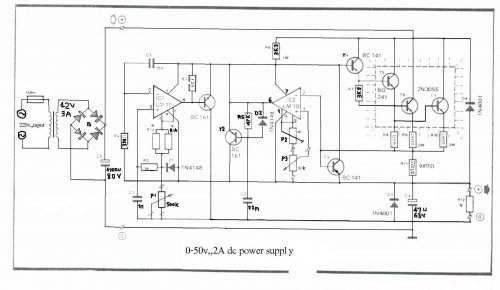# Electronic projects

you are here: home page :: projects :: Power & high voltage :: power supplies :: 0-50V 2A Bench power supply

## 0-50V 2A Bench power supply

Circuit diagramI use the lm10 IC because it has a reference voltage and that’s useful for dc power supply. With two ICs can take different output voltage and amperage. This circuit is protected from short circuit.P2 is for controlling the current at the range of 0-2A. Stabilize the output voltage with R4 on negative pin on op-amp and with R2 & P1 on positive pin.

Op-amp output controls T1 that not let ripple of voltage.T1 increase or decrease ampere of R6 and control the voltage of T5 & T4. Pin 1 is the reference voltage and reference voltage is losing some voltage on R1 that has 100uA . This current passes through P1 too.
Vlose p1=100uA*Rp1
This lose voltage regulate output voltage rate of output current is compare between reference voltage of P3 and lose voltage on R11.T3 is protecting short circuit with R11. For reduce out put voltage to 0v should parallel one resistor 470 ohm in out put. Minimum voltage is 0.4v. The maximum output voltage is fixed with R1b and should not become over of 50v. Therefore your transformer should give 36V, 3A with 4700uF capacitor. T6, T5, T7 need heatsilk.

R1a = 2,2 K
R2 = 10 K
R3, R7 = 3.3 k
R4 = 390 Ohm
R5 = 47 K
R6 = 3.3 K 1Watt
R8 = 180 Ohm
R9, R10 = 0.47 Ohm 3Watt
R11 = 0.075 Ohm 2Watt
R12 = 470 Ohm
P1 = 500K liner potentiometer
P2 = 4.7 K potentiometer
P3 = 10 K potentiometer
C1 = 1nF
C2 = 10nF
C3 = 22nF
C4 = 47mF 63v electrolytic
C5 = 4700mF 80v electrolytic
T1, T2 = BC161
T3, T4 = BD141
T5 = BD241
T6, T7 = 2V3055
D1, D2 = 1N4148
D3, D4 = 1N4001
IC1, IC2 = LM10C

author:
e-mail: hamed_iranmehr@yahoo.com
web site: http://www.electronics-lab.com
project categories:Audio (49)Power & high voltage (43)Radio (23)Light & LED (31)Tools & measurement (40)Telephone (18)Automotive (10)Microcontrollers (12)Sensors & control (47)Timers & oscillators (40)Miscellaneous (26)

StumbleUpon button

project subcategories:
All subcategories
power supplies
battery chargers
high voltage
inverters
free energy
other

similar projects:
10 Amp 13.8 Volt Power Supply
12 Volt 30 Amp PSU
Basic UPS Power Supply
Dual Polarity Power Supply
Dual Regulated Power Supply
Fixed Voltage Power Supply
High Current Power Supply
LM317 VARIABLE POWER SUPPLY
POT-PLANT POWER
Regulated 12 Volt Supply

Doorbell for the Deaf
High Quality Intercom
Combinational Conjuring Trick
POT-PLANT POWER
Ultrasonic Dog Whistle
Quiz Circuit
LED Torch
Insect Repellant
Speaker Microphone Circuit
Magic Wand Conjuring Trick.

most popular projects:
Led display digital Voltmeter
500W low cost 12V to 220V inverter
Low pass filter - Subwoofer
Metal Detector
High And Low Voltage Cut Off With Time Delay
2N3055 Power Amplifier
Basic UPS Power Supply
200W audio amplifier
LED Torch
100W RMS Amplifier

DIY Electronics Projects